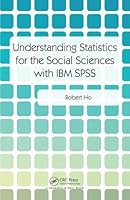# Understanding Statistics for the Social Sciences with IBM SPSS0 Reviews
2017-09-24
296 pages

## Book Description

Modern statistical provides the ability to compute statistics in a timely, orderly fashion. This introductory statistics textbook presents clear explanations of basic statistical concepts and introduces students to the IBM SPSS program to demonstrate how to conduct statistical analyses via the popular point-and-click and the " file" methods. The focal point is to show students how easy it is to analyse data using SPSS once they have learned the basics.

• Provides clear explanation of basic statistical concepts that provides the foundation for the beginner students’ statistical journey.
• Introduces the SPSS software program.
• Gives clear explanation of the purpose of specific statistical procedures (e.g., frequency distributions, measures of central tendencies, measures of variability, etc.).
• Avoids the conventional cookbook approach that contributes very little to students’ understanding of the rationale of how the correct results were obtained.

The advantage of learning the IBM SPSS software package at the introductory class level is that most social sciences students will employ this program in their later years of study. This is because SPSS is one of the most popular of the many statistical packages currently available. Learning how to use this program at the very start not only familiarizes students with the utility of this program but also provides them with the experience to employ the program to conduct more complex analyses in their later years.

Chapter 1. Introduction To The Methodology Of Research
Chapter 2. Introduction To Spss

Section I: Descriptive Statistics
Chapter 3. Basic Concepts And Measurement
Chapter 4. Frequency Distributions
Chapter 5. Graphing
Chapter 6. Measures Of Central Tendency
Chapter 7. Measures Of Variability/Dispersion
Chapter 8. The Normal And Standard Scores
Chapter 9. Correlation
Chapter 10. Linear Regression

Section II: Inferential Statistics
Chapter 11. Statistical Inference And Probability
Chapter 12. Introduction To Hypothesis Testing
Chapter 13. Hypothesis Testing: T Test For Independent And Correlated Groups
Chapter 14. Hypothesis Testing: One-Way Of Variance
Chapter 15. Hypothesis Testing: Chi-Square Test

## Book Details

• Title: Understanding Statistics for the Social Sciences with IBM SPSS
• Author:
• Length: 296 pages
• Edition: 1
• Language: English
• Publisher:
• Publication Date: 2017-09-24
• ISBN-10: 1138742201
• ISBN-13: 9781138742208